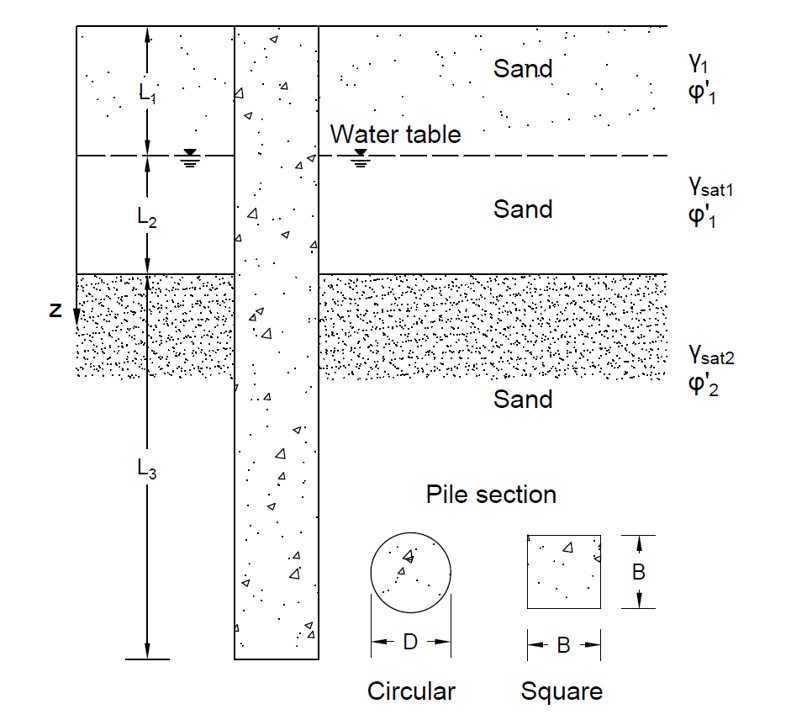# Pile Foundation Tool (sand)## Geometry of Pile Foundation in Sand## Tips

• This tool is for calculating pile foundation bearing capacity in sand, as shown in the figure above.
• Parameter K is used to calculate fricional resistance, for H-piles, K=1.65; for steel pipe piles, K=1.26; for precast concrete piles, K=1.5 (Mansur and Hunter).
• All the input parameters should be positive.
• The unit weight of water is 9.8 kN/m3, and the atmospheric pressure is 100 kPa.
• For "nan", "0" or "inf" displayed in Results, please check your input parameters.
• If Qall is given to estimate Fs, users can try to input different Fs to see the "Qall" in Results. The real Fs corresponds to the caluclated "Qall" same as the given Qall.
• Same logic of trial-error process can be used to estimate the dimension of the pile (diameter & length) for a given Qall and a required Fs.

L1 (m)
L2 (m)
L3 (m)
γ1 (kN/m3)
γsat1 (kN/m3)
γsat2 (kN/m3)
φ′1 (°)
φ′2 (°)
Es (kPa)
ν
K
δ′/φ′
Fs